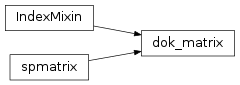# mvpa2.algorithms.group_clusterthr.dok_matrix¶class `mvpa2.algorithms.group_clusterthr.``dok_matrix`(arg1, shape=None, dtype=None, copy=False)

Dictionary Of Keys based sparse matrix.

This is an efficient structure for constructing sparse matrices incrementally.

This can be instantiated in several ways:
dok_matrix(D)
with a dense matrix, D
dok_matrix(S)
with a sparse matrix, S
dok_matrix((M,N), [dtype])
create the matrix with initial shape (M,N) dtype is optional, defaulting to dtype=’d’

Notes

Sparse matrices can be used in arithmetic operations: they support addition, subtraction, multiplication, division, and matrix power.

Allows for efficient O(1) access of individual elements. Duplicates are not allowed. Can be efficiently converted to a coo_matrix once constructed.

Examples

```>>> import numpy as np
>>> from scipy.sparse import dok_matrix
>>> S = dok_matrix((5, 5), dtype=np.float32)
>>> for i in range(5):
...     for j in range(5):
...         S[i, j] = i + j    # Update element
```

Attributes

 `nnz` Number of stored values, including explicit zeros.
 dtype (dtype) Data type of the matrix shape (2-tuple) Shape of the matrix ndim (int) Number of dimensions (this is always 2)

Methods

 `asformat`(format) Return this matrix in a given sparse format `asfptype`() Upcast matrix to a floating point format (if necessary) `astype`(t) `clear`(() -> None.  Remove all items from D.) `conj`() `conjtransp`() Return the conjugate transpose `conjugate`() `copy`() Returns a copy of this matrix. `count_nonzero`() Number of non-zero entries, equivalent to `diagonal`() Returns the main diagonal of the matrix `dot`(other) Ordinary dot product `fromkeys`(...) v defaults to None. `get`(key[, default]) This overrides the dict.get method, providing type checking but otherwise equivalent functionality. `getH`() `get_shape`() `getcol`(j) Returns a copy of column j of the matrix as a (m x 1) DOK matrix. `getformat`() `getmaxprint`() `getnnz`([axis]) Number of stored values, including explicit zeros. `getrow`(i) Returns a copy of row i of the matrix as a (1 x n) DOK matrix. `has_key`((k) -> True if D has a key k, else False) `items`(() -> list of D’s (key, value) pairs, ...) `iteritems`(() -> an iterator over the (key, ...) `iterkeys`(() -> an iterator over the keys of D) `itervalues`(...) `keys`(() -> list of D’s keys) `maximum`(other) `mean`([axis, dtype, out]) Compute the arithmetic mean along the specified axis. `minimum`(other) `multiply`(other) Point-wise multiplication by another matrix `nonzero`() nonzero indices `pop`((k[,d]) -> v, ...) If key is not found, d is returned if given, otherwise KeyError is raised `popitem`(() -> (k, v), ...) 2-tuple; but raise KeyError if D is empty. `power`(n[, dtype]) `reshape`(shape[, order]) Gives a new shape to a sparse matrix without changing its data. `resize`(shape) Resize the matrix in-place to dimensions given by ‘shape’. `set_shape`(shape) `setdefault`((k[,d]) -> D.get(k,d), ...) `setdiag`(values[, k]) Set diagonal or off-diagonal elements of the array. `sum`([axis, dtype, out]) Sum the matrix elements over a given axis. `toarray`([order, out]) Return a dense ndarray representation of this matrix. `tobsr`([blocksize, copy]) Convert this matrix to Block Sparse Row format. `tocoo`([copy]) Convert this matrix to COOrdinate format. `tocsc`([copy]) Convert this matrix to Compressed Sparse Column format. `tocsr`([copy]) Convert this matrix to Compressed Sparse Row format. `todense`([order, out]) Return a dense matrix representation of this matrix. `todia`([copy]) Convert this matrix to sparse DIAgonal format. `todok`([copy]) Convert this matrix to Dictionary Of Keys format. `tolil`([copy]) Convert this matrix to LInked List format. `transpose`([axes, copy]) Reverses the dimensions of the sparse matrix. `update`(([E, ...) If E present and has a .keys() method, does: for k in E: D[k] = E[k] `values`(() -> list of D’s values) `viewitems`(...) `viewkeys`(...) `viewvalues`(...)
`conjtransp`()

Return the conjugate transpose

`copy`()

Returns a copy of this matrix.

No data/indices will be shared between the returned value and current matrix.

`count_nonzero`()

Number of non-zero entries, equivalent to

np.count_nonzero(a.toarray())

Unlike getnnz() and the nnz property, which return the number of stored entries (the length of the data attribute), this method counts the actual number of non-zero entries in data.

`format` = 'dok'
`get`(key, default=0.0)

This overrides the dict.get method, providing type checking but otherwise equivalent functionality.

`getcol`(j)

Returns a copy of column j of the matrix as a (m x 1) DOK matrix.

`getnnz`(axis=None)

Number of stored values, including explicit zeros.

Parameters: axis : None, 0, or 1 Select between the number of values across the whole matrix, in each column, or in each row.

`count_nonzero`
Number of non-zero entries
`getrow`(i)

Returns a copy of row i of the matrix as a (1 x n) DOK matrix.

`resize`(shape)

Resize the matrix in-place to dimensions given by ‘shape’.

Any non-zero elements that lie outside the new shape are removed.

`tocoo`(copy=False)

Convert this matrix to COOrdinate format.

With copy=False, the data/indices may be shared between this matrix and the resultant coo_matrix.

`tocsc`(copy=False)

Convert this matrix to Compressed Sparse Column format.

With copy=False, the data/indices may be shared between this matrix and the resultant csc_matrix.

`todok`(copy=False)

Convert this matrix to Dictionary Of Keys format.

With copy=False, the data/indices may be shared between this matrix and the resultant dok_matrix.

`transpose`(axes=None, copy=False)

Reverses the dimensions of the sparse matrix.

Parameters: axes : None, optional This argument is in the signature solely for NumPy compatibility reasons. Do not pass in anything except for the default value. copy : bool, optional Indicates whether or not attributes of `self` should be copied whenever possible. The degree to which attributes are copied varies depending on the type of sparse matrix being used. p : `self` with the dimensions reversed.

`np.matrix.transpose`